﻿ Experimental Study and CFD Simulation of Two-Phase Flow around Multi-Shape Obstacles in Enlarging Channel

### Experimental Study and CFD Simulation of Two-Phase Flow around Multi-Shape Obstacles in Enlarging Ch...

Laith Jaafer Habeeb, Riyadh S. Al-TuraihiOPEN ACCESSPEER-REVIEWED

## Experimental Study and CFD Simulation of Two-Phase Flow around Multi-Shape Obstacles in Enlarging Channel

Laith Jaafer Habeeb1,, Riyadh S. Al-Turaihi2

1Mechanical Engineering Department, University of Technology, Baghdad, Iraq

2College of Engineering/ Department of Mech. Eng., Babylon University, Babil, Iraq

### Abstract

Experimental and numerical studies are investigated to study the two-phase flow phenomena around multi- shape obstacles in a rectangular enlarging channel which has the dimensions (10 × 3 × 70 cm) enlarged from assembly circular tube of the two phases. Experiments are carried out in the channel with air-water flow with different air and water flow rates. These experiments are aimed to visualize the two phase flow phenomena as well as to study the effect of pressure difference through the channel with the existence of the obstacle. All sets of the experimental data in this study are obtained by using a pressure transducer and visualized by a video camera for different water discharges (20, 25, 35 and 45 l/min) and different air discharges (10, 20, 30 and 40 l/min). While the numerical simulation is conducted by using commercial Fluent CFD software to investigate the steady and unsteady turbulent two dimensional flows for different air and water velocities. The results showed that when air or water discharge increases, high turbulence is appear which generate more bubbles and waves and the mean pressure difference increases. Also, in a water slug, bubbles move slower than the liquid.

### At a glance: Figures

• Habeeb, Laith Jaafer, and Riyadh S. Al-Turaihi. "Experimental Study and CFD Simulation of Two-Phase Flow around Multi-Shape Obstacles in Enlarging Channel." American Journal of Mechanical Engineering 1.8 (2013): 470-486.
• Habeeb, L. J. , & Al-Turaihi, R. S. (2013). Experimental Study and CFD Simulation of Two-Phase Flow around Multi-Shape Obstacles in Enlarging Channel. American Journal of Mechanical Engineering, 1(8), 470-486.
• Habeeb, Laith Jaafer, and Riyadh S. Al-Turaihi. "Experimental Study and CFD Simulation of Two-Phase Flow around Multi-Shape Obstacles in Enlarging Channel." American Journal of Mechanical Engineering 1, no. 8 (2013): 470-486.

 Import into BibTeX Import into EndNote Import into RefMan Import into RefWorks

### 1. Introduction

The understanding of turbulent two-phase bubbly ﬂows is important due to the widespread occurrence of this phenomenon in natural and engineering systems . The flow patterns of gas-liquid two-phase flow could be bubble flow, slug flow, plug flow, stratified flow, wavy flow and disperse flow. There are still many challenges associated with a fundamental understanding of ﬂow patterns in multiphase ﬂow and considerable research is necessary before reliable design tools become available. Gas-liquid flow was extensively used in industrial systems such as power generation units, cooling and heating systems (i.e. heat exchangers and manifolds), safety valves, etc. Thus two-phase flow characteristics through these singularities should be identified in order to be used in designing of the system . In the regimes of bubbly and slug ﬂows, a spectrum of different bubble sizes is observed. While dispersed bubbly ﬂows with low gas volume fraction are mostly mono-dispersed, an increase in the gas volume fraction leads to a broader bubble size distribution due to breakup and coalescence of bubbles. Moreover, the forces acting on the bubbles may depend on their individual size . In the last decade, the stratified flows are increasingly modeled with computational fluid dynamics (CFD) codes. In CFD, closure models are required that must be validated. The recent improvements of the multiphase flow modeling in the ANSYS code make it now possible to simulate these mechanisms in detail . A general estimates for mean velocities through and around groups or arrays of ﬁxed and moving bodies, in unbounded and bounded domains, which lie within a deﬁned perimeter were derived . When the bodies were well-separated, the interstitial Eulerian and Lagrangian mean velocities were deﬁned and calculated in terms of the far-ﬁeld contributions from the velocity or displacement ﬁeld within the group of bodies. The development of numerical code to deal with incompressible two phase flow around 2D hydrofoil combined with cavitation model with k-ε turbulent model was focused . Also, the calculation of cavitation in cryogenic fluid was done by implementing the temperature sensitivity in government equations. Even though the results showed good agreement with previous calculation results, further research will be needed to account for real physics of the formation of cavitation in cryogenic fluid due to temperature sensitivity. The air– water bubbly ﬂow around two tandem square-section cylinders with the vortex method proposed by the authors in a prior study was simulated . Pair of Karman vortices appeared behind the ﬁrst cylinder. The strength of the Karman vortex downstream of the second cylinder was larger than that for the single-cylinder. The strength of the Karman vortex reduced due to the bubble entrainment. The pressure reduction on the rear surface of the ﬁrst cylinder was relaxed when compared with that on the single-cylinder. Therefore, this simulation could predict the reduction of the drag force acting on the ﬁrst cylinder, which was clariﬁed by the corresponding experimental study. Supercavitation around a hydrofoil based on ﬂow visualization and detailed velocity measurement was studied . A high-speed video camera was used to visualize the ﬂow structures under different cavitation numbers, and a particle image velocimetry (PIV) technique was used to measure the instantaneous velocity and vorticity ﬁelds. It was shown that the cavitation structure depends on the interaction of the water–vapor mixture and the vapor among the whole supercavitation stage. As the cavitation number was progressively lowered, three supercavitating ﬂow regimes were observed. Furthermore, in the cavitating region, strong momentum transfer between the higher and lower ﬂow layers taken place, resulting in a highly even velocity distribution in the core part of the cavitating region, and the lower velocity area became smaller and, as the cavitation number lowers, moved toward the downstream. A population balance model in close cooperation between ANSYS-CFX and Forschungszentrum Dresden- Rossendorf and implemented into the CFD-Code CFX was developed . They presented a brief description of the model principles. The capabilities of this model were discussed via the example of a bubbly ﬂow around a half- moon shaped obstacle. For the complex ﬂow around the obstacle, the general structure of the ﬂow was well reproduced in the simulations. This test case demonstrated the complicated interplay between size dependent bubble migration and the effects of bubble coalescence and breakup on real ﬂows. However, clear deviations occur for bubble coalescence and fragmentation. An analytical solution for local pressure drop due to obstructions in horizontal air-water two-phase flow was presented . Various obstruction shapes with size were investigated. The relationship between two-phase multiplier and local (normalized) pressure drop with the gas superficial velocity were investigated. The results showed a higher pressure drops pointed for larger obstructions. A correlation study on the two-phase multipliers and local pressure drop for two-phase, air water mixture flows through obstructions in horizontal channel was made. The numerical simulation to investigate the gas-liquid two- phase flow in the microchannel for the adhesive dispensing was carried out . A 2-D model of microchannel with a diameter for 0.04 mm was established and meshed. The gas-liquid slug flow emerges after iterating over 1 million steps with the gas flow rate for 0.1 m/s, the water flow rate for 0.05 m/s. While the gas flow rate decreased, the length of liquid droplet and gas bubble increased and decreased, respectively and the total numbers of bubble and droplet decreased in one period. This indicated the fluid parameters have a high relationship with the quantity and volume of droplet. The vortex structures and particle dispersions in ﬂows around a circular cylinder by lattice Boltzmann method (LBM), with non-equilibrium extrapolation method (NEM) dealing with the computational boundaries was investigated . It was found that both the Reynolds number and the Stokes number produce signiﬁcant inﬂuences on the particle distribution. The small particles (St = 0.01) follow the motion of the ﬂuid very well and can disperse into the core regions of the vortex structures. The particles at intermediate Stokes numbers (St = 0.1 and 1) concentrate on the boundaries of the vortices, and the large particles (St = 10) also assemble in the outer regions of the vortices under the inﬂuence of the vortex structures. Characteristics of adiabatic air-water flow through a horizontal channel having smooth expansion (enlarging), which was obtained by inspiring from one of the singularities existing in safety valves, were investigated numerically . Numerical simulations are performed via commercial software, GAMBIT (v. 2.3.16) and FLUENT (v. 6.3.26), for the flow upstream, through and downstream the singularity. Eulerian Model was employed for the analysis. Internal diameter of the channel enlarges from 32 mm to 40 mm. Flow rate for water was constant at 3 l/s while that for air was taken as 50 and 61 l/min. The effect of ﬂuid properties and operating conditions on the generation of gas–liquid Taylor ﬂow in microchannels was investigated experimentally and numerically . Visualization experiments and 2D numerical simulations have been performed to study bubble and slug lengths, liquid ﬁlm hold-up and bubble velocities. The results showed that the bubble and slug lengths increase as a function of the gas and liquid ﬂow rate ratios. Numerical studies on the hydrodynamic and heat transfer characteristics of two- phase ﬂows in small tubes and channels were reviewed . The review was then categorized into two groups of studies: circular and non-circular channels. Different aspects such as slug formation, slug shape, ﬂow pattern, pressure drop and heat transfer were of interest. Gaps in research were found in applications of non-circular ducts, pressure drop and heat transfer in meandering microtubes and microchannels for both gas–liquid and liquid–liquid two-phase ﬂows.

From the previous review it is denoted that the recent researches in the two phase flow with the enlargement and existence of a multi- shape cylinders are very limited. So, our concern in this study is to study the effects of wide range of air/water discharge in the steady and unsteady cases on the flow behavior with the enlargement from the circular tube of the water phases which contains the air phase tube, to the rectangular duct with the existence of a circular cylinder. For the experimental investigation of co- current air/water flows, the HAWAC (Horizontal Air/Water Channel) was built. The channel allows in particular the study of air/water slug/disperse flow under atmospheric pressure. Parallel to the experiments, CFD calculations were carried out. The behavior of slug/disperse generation and propagation was qualitatively reproduced by the simulation.

### 2. The Physical Model and Experimental Apparatus

Figure 1 shows a schematic and photograph of the experimental Apparatus and measurements system. The rig is consists of, as shown in Figure 2:

Figure 2. (a) Water system, (b) Air flow meter, (c) Enlargement connecting part, flange, piping system and pressure transducer sensor

1.   Main water tank of capacity (1 m3).

2.   Water pump with specification quantity (0.08 m3/min) and head (8 m).

3.   Valves and piping system (1.25 in)

4.   Adjustable volume flow rate of range (10-80 l/min) is used to control the liquid (water) volume flow rates that enter test section.

5.   Air compressor and it has a specification capacity of (0.5 m3) and maximum pressure of (16 bars).

6.   Rotameter was used to control the gas (air) volume flow rates that enter the test section. It has a volume flow rate range of (6-50 l/min).

7.   Valves and piping system (0.5 in) and gages.

8.   Pressure transducer sensors which are used to record the pressure field with a range of (0-1 bar) and these pressure transducer sensors are located in honeycombs at the entrance and at the end of the test section. The pressure sensors with a distance of (80 cm) between each other are measuring with an accuracy of (0.1%).

9.   The obstacle used is made of stainless steel and its dimensions are (3 cm) for the length of each obstacle and; (3 cm) diameter for the circular cylinder, (3 cm) for the square-section cylinder sides and (3 cm) for each of the triangle sides and, which is coated with a very thin layer of black paint and its center located at (11.5 cm) from the entrance of the test section.

10. The test section is consisting of rectangular channel and a cylindrical obstacle. The rectangular cross sectional area is (10 cm 3 cm) and has length of (70 cm) which is used to show the behavior of the two phase flow around the obstacle and to measure the pressure difference and records this behavior. The obstacle is mounted and fixed by screw and nut on a blind panel on the bottom of the rectangular channel. The three large Perspex windows of the channel (two lateral sides with lighting and the top side) allowing optical access through the test section. Two enlargement connecting parts are made of steel and manufactured with smooth slope. The first on is used to connect the test section with the outside water pipe in the entrance side while the second one is used to connect the test section with the outside mixture pipe in the exit side. The inside air pipe, in the entrance side, is holed and contained inside the water pipe by a steel flange.

11. Interface system consists of two parts which are the data logger and the transformer which contains in a plastic box. The data logger has a three connections two of them are connected to the outside of the box (one connected to the sensors and the other connected to the personal computer), the third connection is connected to the transformer, which is work to receive the signals as a voltage from the sensors and transmit it into the transformer and then re-received these signals after converting it to ampere signals in the transformer.

12. The interface system which is connected with a personal computer so that the measured pressure across the test section is displayed directly on the computer screen by using a suitable program.

13. A Sony digital video camera recorder of DCR- SR68E model of capacity 80 GB with lens of Carl Zeiss Vario-Tessar of 60 x optical, 2000 x digital is used to visualize the flow structure. The visualized data are analyzed by using a AVS video convertor software version 8.1. A typical sequence snapshots recorded by the camera using a recording rate of 30 f/s.

The flows of both gas and liquid are regulated respectively by the combination of valves and by-passages before they are measured by gas phase flow meter and liquid phase flow meter. The gas phase and the liquid phase are mixed in the enlargement connection part before they enter the test section. When the two-phase mixture flows out of the test section, the liquid phase and the gas phase are separated in liquid storage tank. Experiments were carried out to show the effect of different operation conditions on pressure difference across the test section and to visualize the flow around the obstacle. These conditions are water discharges and air discharges. The selected experimental values for water discharges are (20, 25, 35 and 45 l/min) and for air discharges are (10, 20, 30 and 40 l/min). The experimental procedures are:

1. Fix the circular cylinder obstacle at the channel bottom side.

2. Turn on the water pump at the first value (20 l/min).

3. Turn on the air compressor at the first value (10 l/min).

4. Record the pressure drop through the test section and photograph the motion of the two-phase flow by the digital camera.

5. Repeat steps (2 to 5) by changing the water discharge.

6. Repeat step (5) by changing the air discharge.

7. Change the circular cylinder obstacle by the square-section cylinder obstacle and then by the triangular-section cylinder obstacle.

These give sixteen (16) experimental cases for volume fraction (Air/Water ratios) for each type of obstacles.

### 3. Numerical Modelling

In this study, the computational fluid dynamics (CFD) software have been applied for the numerical simulation for adiabatic gas-liquid flow characteristics through a horizontal channel contain a cylinder (different shape every time) with smooth expansion from the liquid pipe in steady, unsteady and 2D cases. In order to compare numerical results with experimental ones, air-water couple has been selected as the representative of the gas-liquid two-phase flow. Construction of the numerical domain and the analysis are performed via GAMBIT and FLUENT (ANSYS 13.0) CFD codes, respectively. Two- phase flow variables such as void fraction and flow velocity for liquid (water) and gas (air) at the inlet condition, and the geometrical values of the system (i.e. channel length, width and height, pipes and inlet enlargement connecting part dimensions, and obstacle dimensions) used in the analysis are selected as the same variables as the experimental part. Atmospheric conditions are valid for the experimental facility. Total test rig length in the experiments, thus in the numerical domain, is (100 cm) including (70 cm) for the test section containing the obstacle, and (30 cm) for the inlet enlargement part. Water pipe diameter is (3.175 cm) and air pipe diameter is (1.27 cm) as shown in Figure 3 (for the circular cross section cylinder).

The 2D physical model is established using a model of flow focusing channel in CFD. The enlargement connecting part length consists of: (0.05 m) circular pipe, m) diverge-link to change the shape from circular to rectangular and (0.1 m) rectangular duct. Air and water are selected to be working fluids and their fluid properties are in Table 1.

#### Table 1. Property parameters of the gas and liquid in CFD

The model geometry structure was meshed by the preprocessor software of GAMBIT with the Quad/Pave grids for the circular obstacle and Quad/Submap grids for square-section and triangular-section obstacles. After meshing, the model contained (for 2D ) 14978 grid nodes and 14522 cells for the circular obstacle, 16282 grid nodes and 32090 faces for square-section obstacle and 14447 grid nodes and 29348 faces for triangular-section obstacle (as demonstrated in Figure 4 for the square cross section cylinder) before importing into the processor Fluent for calculation. This refinement grid provided a precise solution to capture the complex flow field around the cylinder and mixing region in the enlargement connecting part. The boundary conditions are the velocity inlet to the air and water feeding (Table 2) and the pressure outlet to the model outlet. In Fluent, the Mixture and Eulerian Multiphase models were adopted to simulate the flow. The mixture model is a simplified Eulerian approach for modeling n-phase flows, while the Eulerian multiphase model is a result of averaging of NS equations over the volume including arbitrary particles + continuous phase and the result is a set of conservation equations for each phase (continuous phase + N particle “media”) . Because the flow rates of the air and water in the channel are high, the turbulent model (k-ε Standard Wall Function) has been selected for calculation. The other options in Fluent are selected: SIMPLE (Semi-Implicit Method for Pressure-Linked Equations) scheme for the pressure-velocity coupling, PRESTO (pressure staggering option) scheme for the pressure interpolation, Green-Gauss Cell Based option for gradients, First-order Up-wind Differencing scheme for the momentum equation, the schiller-naumann scheme for the drag coefficient, manninen-et-al for the slip velocity and other selections are described in Table 3. The time step (for unsteady case), maximum number of iteration and relaxation factors have been selected with proper values to enable convergence for solution which is about to (0.001) for all parameters.

#### Table 3. Other mixture model selections for Fluent

The hydrodynamics of two-phase flow can be described by the equations for the conservation of mass and momentum, together with an additional advection equation to determine the gas-liquid interface. The two- phase flow is assumed to be incompressible since the pressure drop along the axis orientation is small. For the incompressible working fluids, the governing equations of the Mixture Multiphase Model are as following [16, 17]:

− The continuity equation for the mixture is:(1)

Where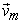is the mass-averaged velocity: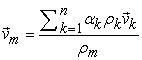(2)

and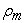is the mixture density:(3)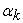is the volume fraction of phase k.

− The momentum equation for the mixture can be obtained by summing the individual momentum equations for all phases. It can be expressed as: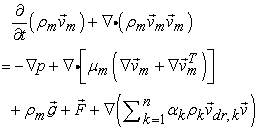(4)

where n is the number of phases,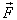is a body force and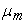is the viscosity of the mixture: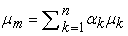(5)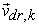is the drift velocity for secondary phase k: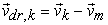(6)

From the continuity equation for secondary phase p, the volume fraction equation for secondary phase p can be obtained: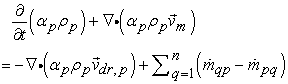(7)

The relative velocity (also referred to as the slip velocity) is defined as the velocity of a secondary phase (p) relative to the velocity of the primary phase (q):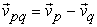(8)

The mass fraction for any phase (k) is defined as: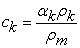(9)

The drift velocity and the relative velocity (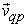) are connected by the following expression: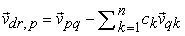(10)

ANSYS FLUENT’s mixture model makes use of an algebraic slip formulation. The basic assumption of the algebraic slip mixture model is that to prescribe an algebraic relation for the relative velocity, a local equilibrium between the phases should be reached over a short spatial length scale. The form of the relative velocity is given by:(11)

whereis the particle relaxation time: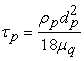(12)

d is the diameter of the particles (or droplets or bubbles) of secondary phase p ,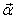is the secondary-phase particle’s acceleration. The default drag function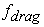:(13)

and the accelerationis of the form: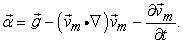(14)

The simplest algebraic slip formulation is the so-called drift flux model, in which the acceleration of the particle is given by gravity and/or a centrifugal force and the particulate relaxation time is modified to take into account the presence of other particles.

In turbulent flows the relative velocity should contain a diffusion term due to the dispersion appearing in the momentum equation for the dispersed phase. ANSYS FLUENT adds this dispersion to the relative velocity: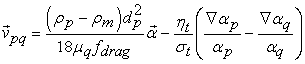(15)

where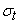is a Prandtl/Schmidt number set to 0.75 and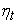is the turbulent diffusivity. This diffusivity is calculated from the continuous-dispersed fluctuating velocity correlation, such that: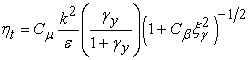(16)(17)

Where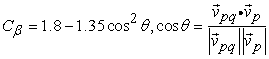and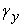is the time ratio between the time scale of the energetic turbulent eddies affected by the crossing-trajectories effect and the particle relaxation time. If the slip velocity is not solved, the mixture model is reduced to a homogeneous multiphase model.

And the governing equations of the Eulerian Multiphase Model are as following [16, 17]:

- The continuity equation is: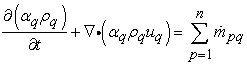(18)

Where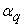is the volume fraction for the qth phase:

- The momentum equation for qth phase: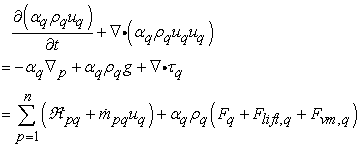(19)

Where the first left hand side term is referring to the transient and the second one is refer to the convection. In the right hand side, the terms are referring to the pressure, body force, shear stress, (interphase forces exchange and interphase mass exchange) and (external, lift, and virtual mass forces) respectively. Hence, solids pressure term is included for granular model. The inter-phase exchange forces are expressed as:(20)

Where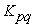is the exchange coefficient and in general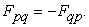In FLUENT application, boundary conditions like “velocity inlet” is taken as the inlet condition for water and air while “interior” and “outflow” are employed as the water-air mixture. Air is injected to the water via an air pipe in the experiments, therefore, the gas flow through the air pipe and the mixture occurred outlet of it are modeled in 3D (Figure 3). According to the simulation, air with known mass flow rate flows through air pipe and then disperses into the water at the exit of the pipe. At air flow rates (thus volumetric void fraction), phase inlet velocity and void fraction profiles obtained at the air and water pipes outlet are extracted from the experimental calculations in order to be introduced as the inlet condition for the flow analysis regarding the numerical 2D domain. In the present study bubble diameter is equal to (1 cm). Assuming the bubbles are in spherical shape and neglecting the coalescence between them along the channel.

### 4. Experimental Results

The experimental results are represented of a gas-liquid flow through channel with the existence of the obstacle for different water discharges (20, 25, 35 and 45 l/min) and different air discharge (10, 20, 30 and 40 l/min) as photographs and as pressure graphs.

4.1. Effect of Air and Water Discharges

Figure 5, Figure 6, Figure 7 and Figure 8 show photographs for the two phase flow behavior around the obstacles for water discharge (Qw=20, 25, 35, 45 l/min) respectively and air discharges (Qa = (a)10, (b)20, (c)30 and (d)40 l/min) for the three cylinders (circular, triangular and square). It shows that the number (amount) of bubble is few and has small size at low water discharge. These photographs describe the flow behavior and it appears that it is near to slug or plug region when the discharge is low. This is due to the low velocity of water at low water discharge. Also when increase the air discharge the size and number of bubbles increases and the bubble cavities develops to cloud cavitations especially at high air discharge. This is due to the high velocity of water at high water discharge which leads to more turbulence in the flow and the flow becomes bubbly as shown. It is clear that the flow becomes unstable and unsymmetrical around the cylinders and the number and size of bubble become higher compared with the low velocities cases. It appears that the vortices behind and beside the cylinders becomes more strong compared with the low discharge cases. When the two phases increases, more unsteady behavior is noticed and the flow oscillates between bubble and disperse regions. Also, when water discharge increases with increase air discharge, flow becomes unsteady, vortices developed around the cylinders surface and most bubbles transformed to cloudy flow, then a disperse region and strong vortex shedding is observed. This is due to the important effect of the cylinders existence in the rectangular channel which effect on pressure difference across the inlet and outlet of the channel. The experimental data shows that the average number of bubbles generally increases with increasing mixture velocities. Independently of the inlet velocities, the highest number of bubbles is found in the mixing region. Moreover, higher gas velocities have a higher number of bubbles in the mixing region and the smooth obstacle (circular cylinder) generates less bubble and turbulence.

4.2. Effect of Pressure Difference

Figure 9 represents the mean pressure difference with air discharge for different values of water discharge for the three cylinders. While Figure 10 represents the mean pressure difference with water discharge for different values of air discharge for the three cylinders. When air or water discharge increases, the mean pressure difference increases. This is due to the increase of air or water discharge resulting in velocity increases. It is already noticed that the circular cylinder has a pressure difference values more than that of the other two obstacles. Also, the mean pressure difference has a significant influence on two-phase flow behavior. Therefore, it is expected that the flow instability will also depend upon the pressure difference.

4.3. Effect of Time Evolution of Pressure

Figure 11 represents effect of time evolution of pressure across the (a) circular, (b) triangular and (c) square cylinder respectively obtained by experiments for water discharge Qw=20 l/min and air discharge Qa=10 l/min at inlet and outlet of the rectangular channel. The pressure sensor at the inlet -after honeycombs- and outlet of the test section are record pressures that fluctuating as a function of time due to two-phase effect.

### 5. Numerical Results

The numerical results are represented as colored contours and center line distributions for the same air- water discharges cases in the experimental part. As mentioned above, the 2D inlet (line) air or water velocities are calculated from the 3D experimental inlet (surface) area from the air or water discharge. These give sixteen (16) numerical cases for volume fraction (Air/Water ratios) for each type of obstacles.

Figures (12-a, b, c, d, f and g) depict volume fraction (water) contours for selected cases (case1, 3, 5, 6, 10, 11 and 12 respectively) for the circular cylinder. While Figures (13-a, b, c, d, e, f and g) depict volume fraction contours for selected cases (1, 2, 4, 5, 11, 12 and 13) respectively for the triangular cylinder. Also, Figures (14-a, b, c, d, e, f and g) depict volume fraction (water) contours for selected cases (case 4, 5, 6, 7, 10, 14and 15 respectively) for the square cylinder. The differences between the experimental snapshots and numerical Figures are due to two reasons; the first is the differences in the overall flow rates of air and water for the same inlet velocities from the inlet regions (small lines in 2D numerical cases and big square and annulus areas in 3D experimental cases), and the second reason is that the snapshots are taken roughly from the experimental movies for each case and may be for another snapshot from the same case movie, the differences will be less. From these figures it is appear that a slug to disperse regions flow pattern is achieved. The flow rates of air and water have a large range and it show the increase in water phase and with the decrease of the gas flow rate, the volume fraction of the gas decreased and the volume fraction of the water increased simultaneously. According to the figures, stratified water-air mixture enters the singularity section and begins to decelerate due to the smoothly enlarging cross-section and it show how the volume fraction affected the flow behavior. A uniform dispersed two-phase flow, in which the dispersed phase (either air bubbles or water droplets) moves with their carrier fluid (water or air), approaches to a circular obstacle. Due to strong changes of both magnitude and direction of local velocities of the fluid flow (i.e. local fluid velocity gradients) and density difference between the dispersed phase and the fluid, the local phase distribution pattern changes markedly around the obstacle. Strong air flows are induced and a strong vortex is created as a result of the entered air and small vortices are also produced. A recirculation zone in the wake, a ﬂow separation at the edge of the obstacle and a wavy motion are noticed. It is appear that maximum turbulent viscosity and high turbulence regions depends on the volume fraction ratio. Also, when air velocity increases, separation area is detected after the cylinder.

Figures (15-a, b, c, d, e and f) represent volume fraction (water) contours development for randomly selected unsteady case8 for the circular cylinder. Figures (16-a, b, c, and d) represent volume fraction (water) contours development for selected unsteady case3 for the triangular cylinder. Figures (17-a, b, c, d, e and f) represent volume fraction (water) contours development for randomly selected unsteady case1 for the square cylinder. It show how the volume fraction develops with time. As can be seen, there are two unsteady asymmetrical pattern recirculating zones behind the cylinder when the volume fraction increases or when the two-phase velocity (Reynolds number) increases.

Figures (18-a, b, c, d, e, f, g, h, i, j, k and l) represent center line (x=0.05 m) of the static and dynamic pressure distributions of the mixture along the test rig (1 m) for unsteady case8 at different time steps for the circular cylinder. It show how the pressures are fluctuating along the channel and the fluctuation is increases with time due to the effects of mixing, two-phase flow, circular cylinder existence and density difference. Region that the line does not passing through it (i.e. empty at position (z) = 0.115 m) is the circular cylinder area, as well as at the beginning (z = -0.3 to z=-0.28 cm) there is no mixing.

Figure 18. Static and dynamic pressure distributions of the mixture along the test rig (1 m) for unsteady case8 at different time steps

### 6. Conclusions

The study has focused on phase distributions in low quality dispersed two phase flows around obstacle. It consists of a theoretical part of a more general nature and an experimental part highlighting bubbly flows around a cylinder in horizontal channel. Concluding remarks are summarized below-

1. A novel approach for fluid dispensing with high consistency and accuracy had been proposed based on the fluid dynamics of the gas-liquid two-phase flow.

2. Random-like manner, inducing pressure, velocity and phase fraction fluctuations: the flow is unsteady, even when the flow rates of gas and liquid are kept constant at the channel inlet.

3. When air discharge increases, high turbulence is appear which generate more bubbles and waves.

4. The pressure sensor at the inlet and outlet of the test section are record pressures that fluctuating as a function of time due to two-phase effect. Also, when air or water discharge increases, the mean pressure difference increases.

5. Due to strong changes of both magnitude and direction of local velocities of the fluid flow and density difference between the dispersed phase and the fluid, the local phase distribution pattern changes markedly around the obstacle.

6. It should be noted that the prediction on the bubble size does not correctly describe the size observed in experiments. This is due to the difference in the numerical definition of vapor bubble and visual bubble boundary.

7. In a water slug, bubbles move slower than the liquid. The average velocity of the bubbles is slightly slower than the slug tail velocity. This means that the dispersed bubbles in the liquid slug will be caught up by the arriving elongated bubble.

8. Realistic bubble trajectories, with a number of bubble trajectories entering the wake of a cylinder, are only obtained if the effect of liquid velocity fluctuations (or turbulence in the liquid) is simulated and some kind of sliding phenomenon for colliding bubbles is taken into account.

9. The effect of the existence of a cylinder is clear in dividing the two-phase flow, generate vortices and finally enhance mixing and the smooth obstacle (circular cylinder) generates less bubble and turbulence.

In this study, diameter of the bubbles is considered constant and coalescence between the bubbles is neglected. However, bubbles in the actual flow break down and unite as the flow develops along the channel and this gives a varying diameter distribution which causes lift and drag forces to be calculated locally. Therefore, a simulation considering the effects of differing bubble diameter and interfacial forces is suggested for better modeling of the flow investigated.

### Nomenclature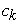: Mass fraction (-): Diameter of the particles (m): Body force (N)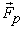: External force (N): Drag function (-)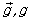: Gravity acceleration (m/s2): Exchange coefficient (-): Mass flow rate (kg/m3.s): Interphase mass exchange (kg/m3.s): Number of phases (-)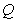: Flow discharge (l/min): Interphase forces exchange (kg/m2.s2)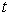: Time(sec)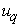: Velocity for the qth phase (m/s)

Greek Symbols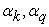: Volume fraction of phase k, q (-): Secondary-phase p (m/s2): Turbulent diffusivity article’s acceleration (N/m2.s): Turbulent diffusivity (kg/m3): Mixture density for the qth phase (kg/m3): Prandtl/Schmidt number (-)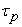: Particle relaxation time (sec)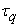: Shear stress for the qth phase (N/m2): Mass-averaged velocity (-): Drift velocity for secondary phase k (velocity of an algebraic slip component relative to the mixture) (-): Viscosity of the mixture (N/m2.s)

Subscripts

a: Air

life: Lift force

k,p,q: Secondary phase

m: Mixture

vm: Virtual mass force

w: Water

### References

  Igor A. Bolotnov, Kenneth E. Jansen, Donald A. Drew, Assad A. Oberai, Richard T. Lahey Jr. and Michael Z. Podowski, “Detached Direct Numerical Simulations of Turbulent Two-Phase Bubbly Channel Flow”, International Journal of Multiphase Flow, 37, (2011), 647-659.In article CrossRef  Brennen and Christopher Earls, “Fundamentals of Multiphase Flow”, Cambridge University Press, 2005.In article  Eckhard Krepper, Matthias Beyer, Thomas Frank, Dirk Lucas and Horst-Michael Prasser, “CFD Modelling of Polydispersed Bubbly Two-Phase Flow Around an Obstacle”, Nuclear Engineering and Design 239 (2009) 2372-2381.In article CrossRef  Thomas HÖHNE, “Experiments and Numerical Simulations of Horizontal Two Phase Flow Regimes”, Seventh International Conference on CFD in the Minerals and Process Industries, CSIRO, Melbourne, Australia, December 2009.In article  I. EAMES, J. C. R. HUNT and S. E. BELCHER, “Inviscid Mean flow through and Around Groups of Bodies”, J. Fluid Mech., vol. 515, pp. 371-89, Cambridge University Press, 2004.In article  Seyoung Lee, Changjin Lee, and Soohyung Park, “Unsteady Cavitation and Cryogenic Flow Cavitation around 2D Body”, IEEE computer society, Fifth International Conference on Computational Science and Applications, 49. pp. 306-312, 2007.In article  T Degawa and T Uchiyama, “Numerical Simulation of Bubbly Flow Around Two Tandem Square-Section Cylinders by Vortex Method”, J. Mechanical Engineering Science, Proc. IMechE Vol. 222 Part C, 2008, pp. 225-234.In article  Xiangbin Li, Guoyu Wang, Mindi Zhang and Wei Shyy, “Structures of Supercavitating Multiphase Flows”, International Journal of Thermal Sciences 47 (2008), pp. 1263-1275.In article CrossRef  Eckhard Krepper, Matthias Beyer, Thomas Frank, Dirk Lucas and Horst-Michael Prasser, “CFD Modelling of Polydispersed Bubbly Two-Phase Flow Around an Obstacle”, Nuclear Engineering and Design 239 (2009) 2372-2381.In article CrossRef  Hameed Balassim Mahood, Hala A. Kadim and Ali N. Salim, “Effect of Flow-Obstruction Geometry on Pressure Drops in Horizontal Air-Water Two-Phase Flow”, Al-Qadisiya Journal For Engineering Sciences, Vol. 2, No. 3, 2009, pp. 641-653.In article  Peng Peng, Jianhua Zhang and Jinsong Zhang, “Simulations for a Novel Fluid Dispensing Technology Based on Gas-liquid Slug Flow”, International Conference on Electronic Packaging Technology & High Density Packaging, IEEE 2011.In article  Hao Zhou, Guiyuan Mo, Kefa Cen, “Numerical Investigation of Dispersed Gas–Solid Two-Phase flow Around a Circular Cylinder Using Lattice Boltzmann Method”, Computers & Fluids, vol. 52, pp. 130-138, 2011.In article CrossRef  Emrah Deniz and Nurdil Eskin, “Numerical Analysis of Adiabatic Two-Phase Flow through Enlarging Channel”, Istanbul Technical University, Mechanical Engineering Faculty, Istanbul, Turkiye, 2011.In article  Thomas Abadie, Joëlle Aubin, Dominique Legendre, Catherine Xuereb, “Hydrodynamics of Gas–Liquid Taylor Flow in Rectangular Microchannels”, Springer-Verlag, Microfluid Nanofluid (2012) 12:355-369.In article CrossRef  V. Talimi, Y. S. Muzychka, S. Kocabiyik, “A Review on Numerical Studies of Slug flow Hydrodynamics and Heat Transfer in Microtubes and Microchannels”, International Journal of Multiphase Flow, vol. 39, pp. 88-104, 2012.In article CrossRef  Introductory FLUENT Notes, FLUENT v6.3, Fluent User Services Center, December 2006.In article  ANSYS 13.0 Help, FLUENT Theory Guide, Mixture Multiphase Model.In article  Esam M. Abed and Riyadh S. Al-Turaihi, “Experimental Study of Two-Phase Flow around Hydrofoil in Open Channel”, Journal for Mechanical and Materials Engineering, Iraq, 2012, accepted and submitted for publication.In article  Riyadh S. Al-Turaihi, “Experimental Investigation of Two-Phase Flow (Gas –Liquid) Around a Straight Hydrofoil in Rectangular Channel”, Journal of Babylon University, Iraq, 2012, accepted and submitted for publication.In article